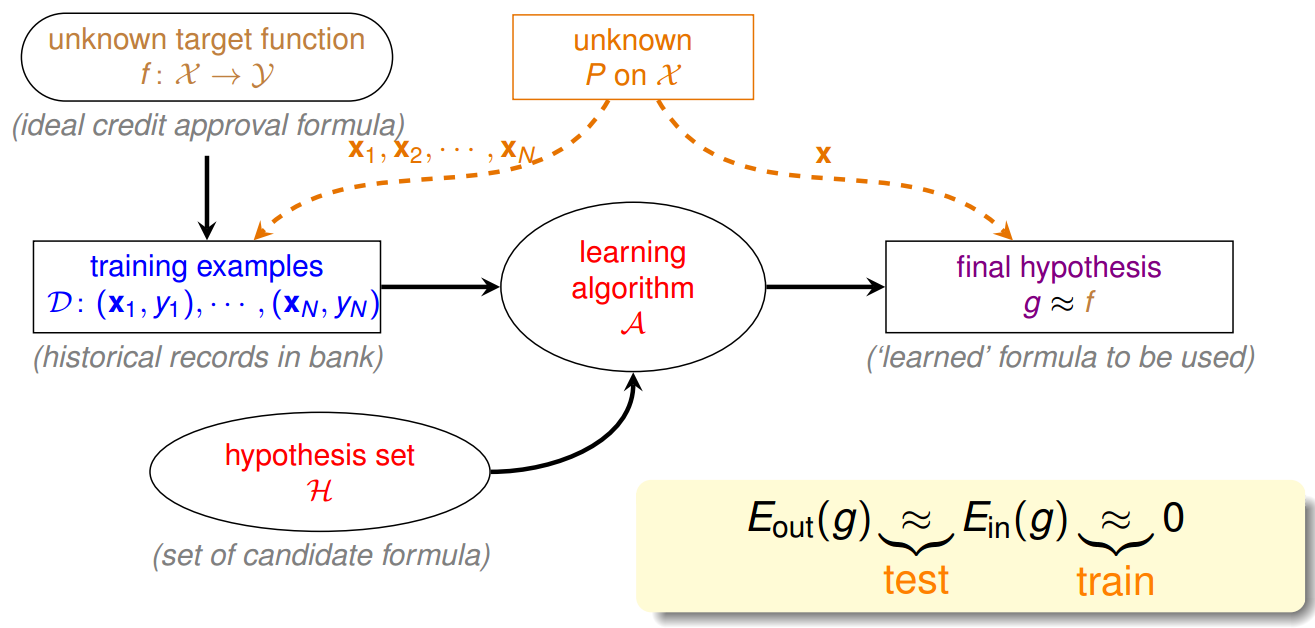## Hoeffding 不等式

$$p(|\nu - \mu| \gt \epsilon) \le 2\exp(-2\epsilon^2N)$$

## 从 Hoeffding 不等式到机器学习

$$p(|E_{in}(h) - E_{out}(h)| \gt \epsilon) \le 2\exp(-2\epsilon^2N)$$

In this framework, the learner receives samples and must select a generalization function (called the hypothesis) from a certain class of possible functions. The goal is that, with high probability (the “probably” part), the selected function will have low generalization error (the “approximately correct” part).

## 从一个 hyposthesis 到多个 hypothesis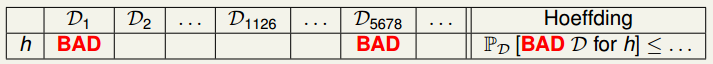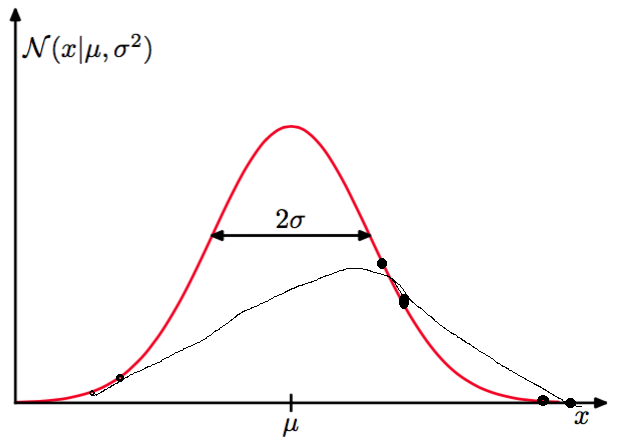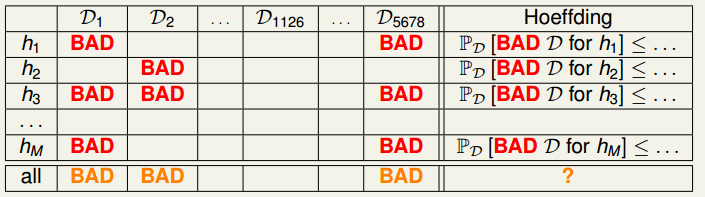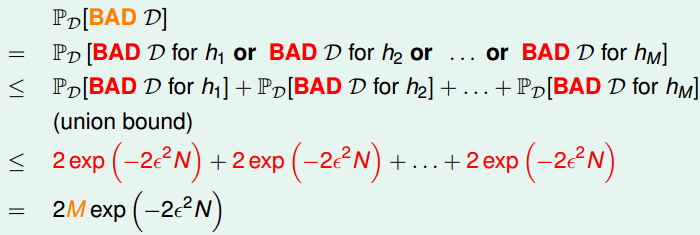## 小结